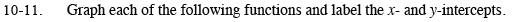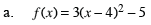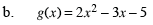Home > CCA2 > Chapter 10 > Lesson 10.1.1 > Problem10-11

10-11.
1. Graph each of the following functions and label the x- and y-intercepts. Homework Help ✎

1. f(x) = 3(x − 4)2 − 5

2. g(x) = 2x2 − 3x − 5The vertex of this parabola is at (4, −5).
Make a table to accurately sketch the graph.

To find the x-intercepts, solve the equation
0 = 3(x − 4)2 − 5.$\text{The }x\text{-coordinate of the vertex of this parabola}$
$\text{is at }x=-\frac{b}{2a}.$